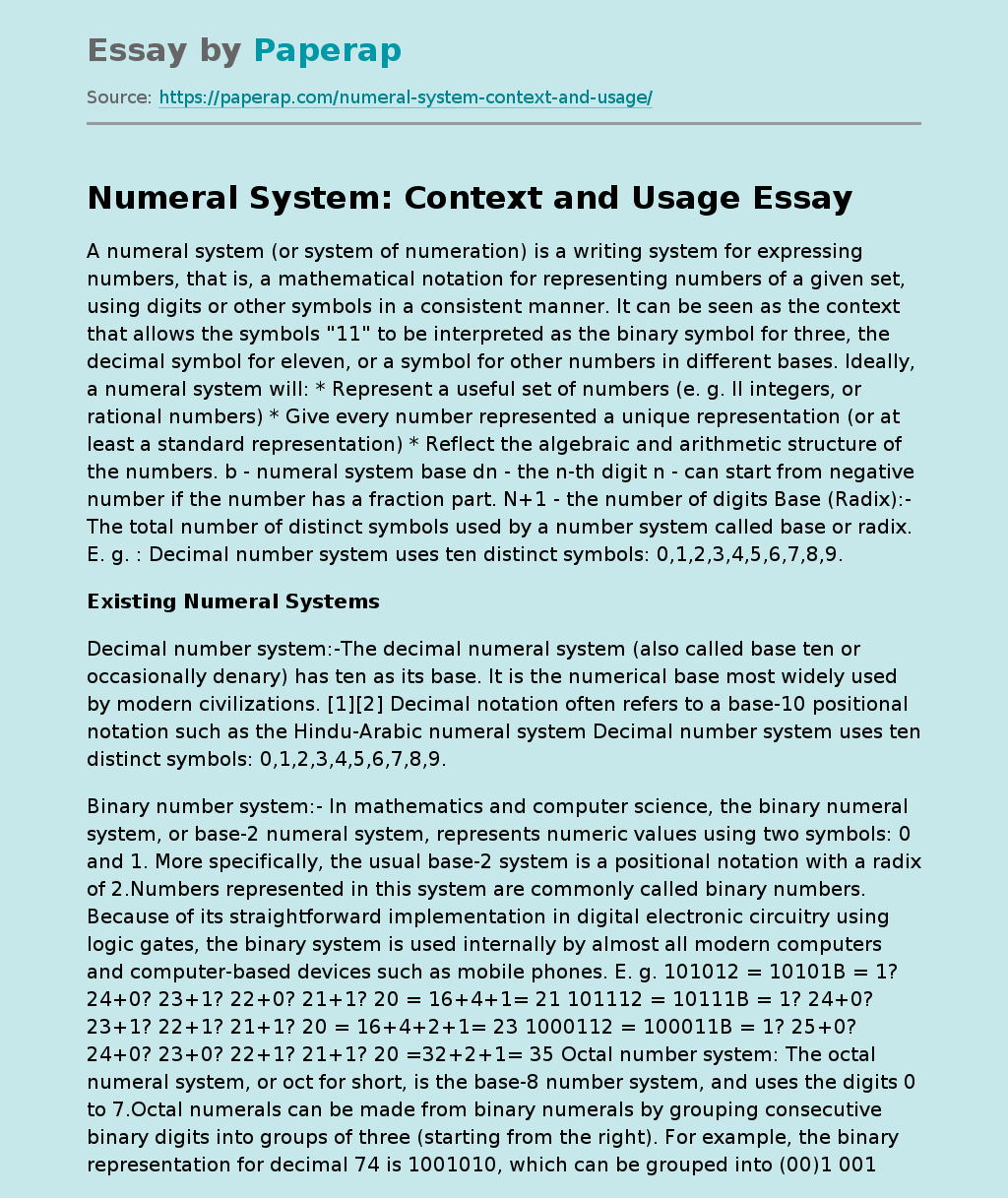# Numeral System: Context and Usage

A numeral system (or system of numeration) is a writing system for expressing numbers, that is, a mathematical notation for representing numbers of a given set, using digits or other symbols in a consistent manner. It can be seen as the context that allows the symbols “11” to be interpreted as the binary symbol for three, the decimal symbol for eleven, or a symbol for other numbers in different bases. Ideally, a numeral system will: * Represent a useful set of numbers (e.

g. ll integers, or rational numbers) * Give every number represented a unique representation (or at least a standard representation) * Reflect the algebraic and arithmetic structure of the numbers. b – numeral system base dn – the n-th digit n – can start from negative number if the number has a fraction part. N+1 – the number of digits Base (Radix):- The total number of distinct symbols used by a number system called base or radix. E. g. : Decimal number system uses ten distinct symbols: 0,1,2,3,4,5,6,7,8,9.

## Existing Numeral Systems

Decimal number system:-The decimal numeral system (also called base ten or occasionally denary) has ten as its base. It is the numerical base most widely used by modern civilizations.  Decimal notation often refers to a base-10 positional notation such as the Hindu-Arabic numeral system Decimal number system uses ten distinct symbols: 0,1,2,3,4,5,6,7,8,9.

Binary number system:- In mathematics and computer science, the binary numeral system, or base-2 numeral system, represents numeric values using two symbols: 0 and 1. More specifically, the usual base-2 system is a positional notation with a radix of 2.

Get quality help nowDr. Karlyna PhDVerified

Proficient in: Communication4.7 (235)

“ Amazing writer! I am really satisfied with her work. An excellent price as well. ”+84 relevant experts are online

Numbers represented in this system are commonly called binary numbers. Because of its straightforward implementation in digital electronic circuitry using logic gates, the binary system is used internally by almost all modern computers and computer-based devices such as mobile phones. E. g. 101012 = 10101B = 1? 24+0? 23+1? 22+0? 21+1? 20 = 16+4+1= 21 101112 = 10111B = 1? 24+0? 23+1? 22+1? 21+1? 20 = 16+4+2+1= 23 1000112 = 100011B = 1? 25+0? 24+0? 23+0? 22+1? 21+1? 20 =32+2+1= 35 Octal number system: The octal numeral system, or oct for short, is the base-8 number system, and uses the digits 0 to 7.Octal numerals can be made from binary numerals by grouping consecutive binary digits into groups of three (starting from the right). For example, the binary representation for decimal 74 is 1001010, which can be grouped into (00)1 001 010 – so the octal representation is 112. In the decimal system each decimal place is a power of ten. For example: In the octal system each place is a power of eight. For example: By performing the calculation above in the familiar decimal system we see why 112 in octal is equal to 64+8+2 = 74 in decimal. E. g. 278 = 2? 1+7? 80 = 16+7 = 23 308 = 3? 81+0? 80 = 24 43078 = 4? 83+3? 82+0? 81+7? 80 = 2247